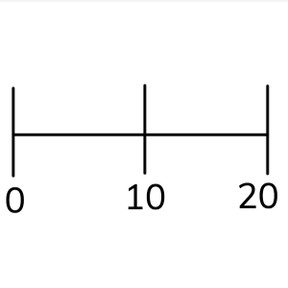Numbers to 20 on the number line

# Numbers to 20 on the number line

Numbers to 20 on the number line8,000 schools use Gynzy92,000 teachers use Gynzy1,600,000 students use Gynzy

## General

Students learn to place numbers to 20 on the number line. They count forwards and backwards and learn that every line represents one number.

K.CC.A.1
K.CC.A.2

## Relevance

Discuss with students that it is important to be able to place numbers on a number line so you know where a number belongs. They can use this to determine which number is more or less.

## Introduction

The interactive whiteboard shows a counting sequence. Hand out number cards to 20 to the students. A few numbers are missing. Ask the students which numbers are missing and to hold up the number card of the missing numbers. Next students are asked to drag the numbers to the correct places in the counting sequence.

## Development

Explain what a number line is and make a number line by making a physical number line (with number cards and a clothes line) or by drawing one on the interactive whiteboard. Tell students that the lowest number always is at the start, so the left side, that every line represents one number, and that the numbers are in the same order as the counting sequence. Discuss with students that you always count forwards from left to right, and that you always count backwards by counting right to left. Point at the numbers as you do so, and then have the students count out loud with you.

Check that students understand number lines and numbers to 20 on the number line by asking the following questions:
What is a number line?
How do you put a number on the number line?
Where do you start counting?
When do you count forward and when do you count back?

## Guided Practice

Students practice placing numbers on a number line where they need to count forwards or backwards to their number. They practice by first selecting from multiple answers, and finally move to determining the answer on their own.

## Closing

Discuss with students that it is important to be able to place numbers on the number line because then you know where a number belongs. Check that students understand what a number line is and have them explain how they determine which numbers are asked for on the number line shown on the interactive whiteboard.

## Teaching Tip

Students who have difficulty placing numbers on the number line can be helped by practicing counting forwards and backwards to 20. They might also be helped by the use of manipulatives.

## Instruction materials

Number cards to 20.

### The online teaching platform for interactive whiteboards and displays in schools

• Save time building lessons

• Manage the classroom more efficiently

• Increase student engagement